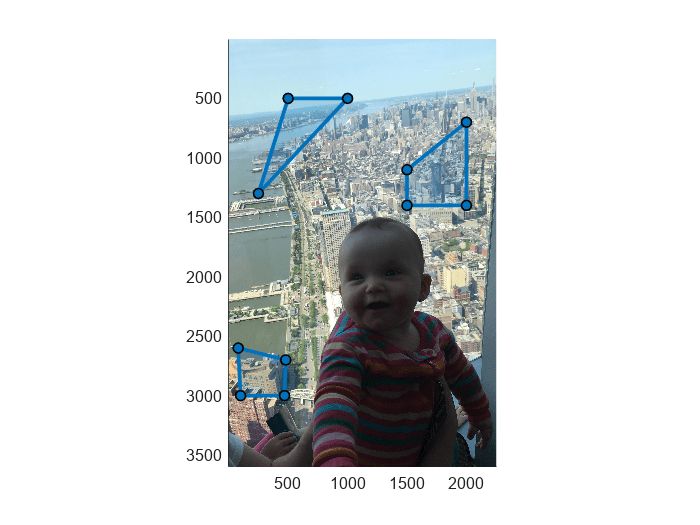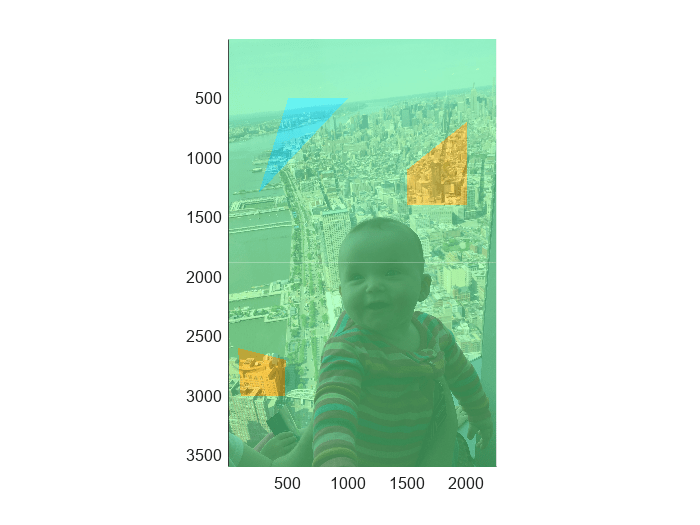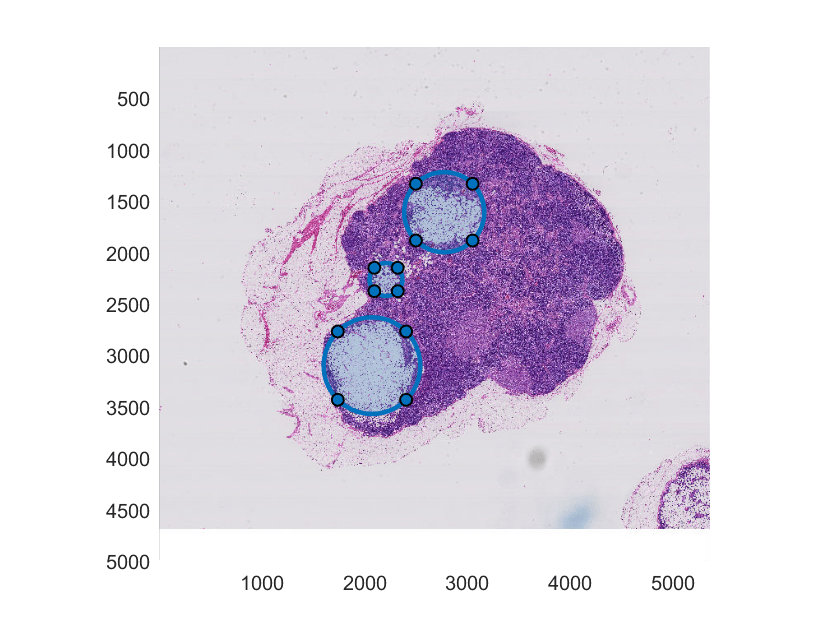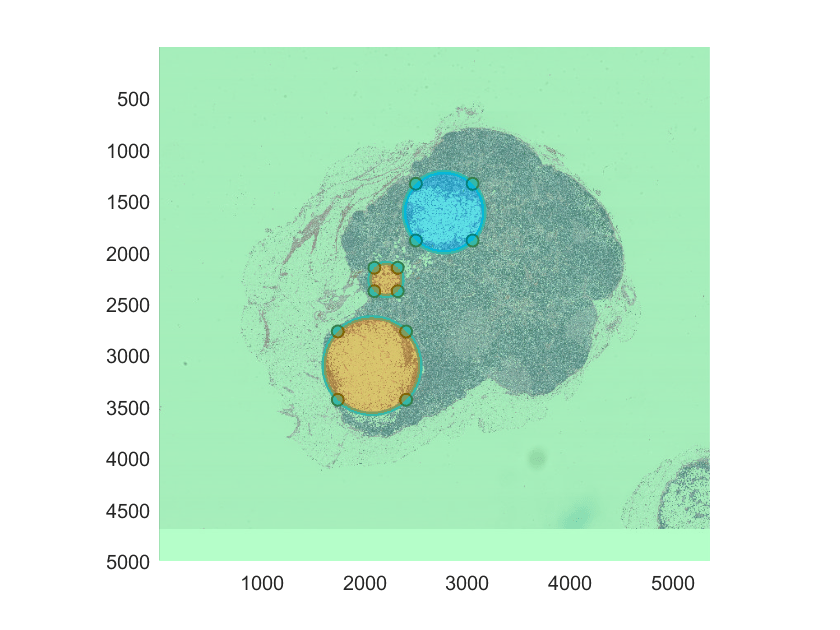# polyToBlockedImage

Create labeled `blockedImage` object from set of ROIs

Since R2021b

## Syntax

``Bout = polyToBlockedImage(ROIpositions,ROILabelIDs,imageSize)``
``Bout = polyToBlockedImage(___,Name=Value)``

## Description

example

````Bout = polyToBlockedImage(ROIpositions,ROILabelIDs,imageSize)` creates a numeric, labeled 2-D blocked image `Bout` of the specified size `imageSize` from the regions of interest (ROIs) defined in `ROIpositions` and label IDs defined in `ROILabelIDs`.```

example

````Bout = polyToBlockedImage(___,Name=Value)` specifies object properties of `Bout` using name-value arguments in addition to the input arguments from the previous syntax.Example: `polyToBlockedImage(ROIpositions,ROILabelIDs,BlockSize=[512,512])` creates a blocked image with a block size of 512-by-512 pixels.```

## Examples

collapse all

Create a blocked image.

`bim = blockedImage("baby.jpg");`

Initialize the ROI position cell array.

```numPolygon = 3; roiPositions = cell(numPolygon,1);```

Specify the coordinates of three ROIs in the `roiPositions` cell array. The first ROI is a triangle, requiring `x`,`y` coordinates for three vertices. The other two ROIs are quadrilaterals, requiring `x`,`y` coordinates for four vertices.

```roiPositions{1} = [500 500; 250 1300; 1000 500]; roiPositions{2} = [1500 1100; 1500 1400; 2000 1400; 2000 700]; roiPositions{3} = [80 2600; 480 2700; 470 3000; 100 3000];```

Display the blocked image, and draw the three ROIs on the figure.

```bigimageshow(bim); for id = 1:numPolygon drawpolygon(Position=roiPositions{id}); end```Create an array for label IDs the same size as the `roiPositions` cell array.

`roilabelID = zeros(numPolygon,1,"uint8");`

Specify label ID values that correspond to the order in which you listed the ROIs in `roiPositions`. The first ROI is a triangle, so label it `1`. The other two ROIs are quadrilaterals, so label them `2`.

```roilabelID(1) = 1; roilabelID(2) = 2; roilabelID(3) = 2;```

Specify the size of the new labeled blocked image to match the size of the initial blocked image.

`imageSize = bim.Size(1:2);`

Create a labeled `blockedImage` object from the ROIs.

`L = polyToBlockedImage(roiPositions,roilabelID,imageSize);`

Display the labeled blocked image. Use color scaling and color axis limits to visualize the differences between the labels for triangular and quadrilateral ROIs.

```bigimageshow(L,CDataMapping="scaled") clim([0 2])```Display the labeled blocked image overlaid on the original blocked image.

```hbim = bigimageshow(bim); showlabels(hbim,L)```Create a blocked image.

`bim = blockedImage("tumor_091R.tif");`

Initialize the ROI position cell array.

```numPolygon = 3; roiPositions = cell(numPolygon,1);```

Specify the center and radius parameters for three circular ROIs.

```center = [2774 1607; 2071 3100; 2208 2262]; radius = [390; 470; 161];```

Display the blocked image, and use `drawcircle` to draw the three circular ROIs on the figure`.` Add the `x`,`y` coordinates of the vertices for each ROI to the `roiPositions` cell array.

```hbim = bigimageshow(bim); for id = 1:numPolygon hROI = drawcircle(Radius=radius(id),Center=center(id,:)); roiPositions{id} = hROI.Vertices; end```Create an array for label IDs the same size as the `roiPositions` cell array.

`roilabelID = zeros(numPolygon,1,"uint8"); `

Specify label ID values that correspond to the order in which you listed the ROIs in `roiPositions`. You can assign each ROI a different label, or group multiple ROIs under the same label.

```roilabelID(1) = 1; roilabelID(2) = 2; roilabelID(3) = 2;```

Specify an image size for the new labeled blocked image equal to that of the second resolution level of the initial blocked image, `bim`.

```maskLevel = 2; imageSize = bim.Size(maskLevel,1:2);```

Create a labeled `blockedImage` object from the ROIs. Use the `WorldStart` and `WorldEnd` name-value arguments to maintain the same world coordinates as the initial blocked image at the specified resolution level.

```L = polyToBlockedImage(roiPositions,roilabelID, ... imageSize,WorldStart=bim.WorldStart(maskLevel, 1:2), ... WorldEnd=bim.WorldEnd(maskLevel, 1:2));```

Display the labeled blocked image overlaid on the original blocked image.

`showlabels(hbim,L)`## Input Arguments

collapse all

Coordinate vectors, specified as a P-element cell array of numeric vectors, where P is the total number of ROIs. Each cell array element is an s-by-2 coordinate vector of the form ```[x1 y1; … ; xs ys]```, where s is the total number of vertices for that ROI. Each x,y pair defines a vertex of the ROI. If the ROI shape is not already closed, the `polyToBlockedImage` function closes the shape automatically. You can specify any number of ROIs.

Data Types: `cell`

Labels for each ROI, specified as a P-element numeric vector or P-element logical vector, where P is the total number of ROIs.

`polyToBlockedImage` assigns the value `0` to all background pixels in the output image.

Data Types: `single` | `double` | `int8` | `int16` | `int32` | `uint8` | `uint16` | `uint32` | `logical`

Size of the output labeled blocked image, specified as a numeric vector of positive integers. If you specify more than two dimensions, the `polyToBlockedImage` function uses only the first two dimensions.

Data Types: `single` | `double` | `int8` | `int16` | `int32` | `uint8` | `uint16` | `uint32`

### Name-Value Arguments

Specify optional pairs of arguments as `Name1=Value1,...,NameN=ValueN`, where `Name` is the argument name and `Value` is the corresponding value. Name-value arguments must appear after other arguments, but the order of the pairs does not matter.

Example: `polyToBlockedImage(ROIpositions,ROILabelIDs,imageSize,BlockSize=[512,512])` creates a labeled blocked image with a block size of 512-by-512 pixels.

Read and write interface for the blocked image object, specified as one of these adapter objects.

`BINBlocks`Store each block as a binary file in a folder
`GenericImage` Store blocks in a single image
`GenericImageBlocks`Store each block as an image file in a folder
`H5`Store blocks in a single HDF5 image
`H5Blocks`Store each block as an HDF5 file in a folder
`InMemory`Store blocks in a variable in main memory
`JPEG2000`Read blocks of a single JPEG2000 file (since R2023a)
`JPEGBlocks`Store each block as a JPEG file in a folder
`MATBlocks`Store each block as a MAT file in a folder
`PNGBlocks`Store each block as a PNG file in a folder
`TIFF`Store blocks in a single TIFF file

You can also create your own adapter using the `images.blocked.Adapter` class.

If `OutputLocation` is specified, then the function automatically selects an adapter based on the output location. If `OutputLocation` is not specified, then the default adapter is an `InMemory` object.

Block size, specified as a numeric vector of positive integers. If you specify more than two dimensions, the `polyToBlockedImage` function uses only the first two dimensions.

Data Types: `double`

Wait bar display toggle, specified as a numeric or logical `1` (`true`) or `0` (`false`). When set to `true`, the `polyToBlockedImage` function displays a wait bar for long-running operations. If you cancel the wait bar, the `polyToBlockedImage` function returns a partial output, if available.

Data Types: `logical`

Location to store the output labeled blocked image, specified as a character vector or string scalar. By default, the output blocked image is stored in memory.

Destination TypeImage Format
Folder name (without a file extension)

The `polyToBlockedImage` function creates the folder and stores blocks of data as files within the folder.

• For numeric image data, `polyToBlockedImage` stores each block as a binary file using the `BINBlocks` adapter.

• For categorical and structure image data, `polyToBlockedImage` stores each block as a MAT file in the folder using the `MATBlocks` adapter.

File name with TIF or TIFF file extension

The `polyToBlockedImage` function stores data as a single TIFF image using the `TIFF` adapter.

The `initialValue` must be numeric or logical with data type `uint8`, `int8`, `uint16`, `int16`, `uint32`, `int32`, `single`, `double` or `logical`.

File name with H5 file extension

The `polyToBlockedImage` function stores data as a single HDF5 image using the `H5` adapter.

The `initialValue` must be numeric with data type `uint8`, `int8`, `uint16`, `int16`, `uint32`, `int32`, `single`, or `double`.

`[]`

The `blockedImage` object stores data as a variable in memory using the `InMemory` adapter.

To specify a custom adapter for other output formats, use the `Adapter` property.

World coordinates of the starting edge of the image, specified as a numeric vector. By default, the value is `[0.5,0.5]`.

Data Types: `double`

World coordinates of the ending edge of the image, specified as a numeric vector. By default, the value is `imageSize + 0.5`, resulting in pixels that are one unit wide.

Data Types: `double`

## Output Arguments

collapse all

Numeric labeled 2-D blocked image, returned as a `blockedImage` object.

## Tips

• To create a labeled blocked image, `Bout`, to overlay on an existing blocked image, match `imageSize` to the size of the existing blocked image at the desired resolution level. If the resolution level of `Bout` matches the finest resolution level of the existing blocked image, you can use the default values for `WorldStart` and `WorldEnd`. To display the overlay at a coarse resolution level, specify `WorldStart` and `WorldEnd` to match the world extents of the existing blocked image at the desired resolution level.

• Creating the labeled blocked image at a coarser resolution level decreases the memory required to store the new blocked image, but decreases the smoothness of the ROI edges.

• If a pixel is inside multiple overlapping ROIs, the function assigns the pixel the label corresponding to the overlapping ROI with the lowest index in `ROIpositions`.

## Version History

Introduced in R2021b